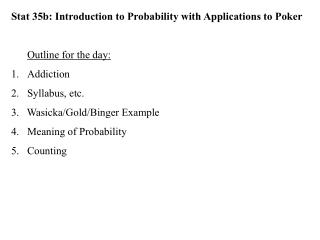# Stat 35b: Introduction to Probability with Applications to Poker Outline for the day: Addiction - PowerPoint PPT PresentationDownload PresentationStat 35b: Introduction to Probability with Applications to Poker Outline for the day: Addiction

Loading in 2 Seconds...

Stat 35b: Introduction to Probability with Applications to Poker Outline for the day: AddictionDownload Presentation## Stat 35b: Introduction to Probability with Applications to Poker Outline for the day: Addiction

- - - - - - - - - - - - - - - - - - - - - - - - - - - E N D - - - - - - - - - - - - - - - - - - - - - - - - - - -
##### Presentation Transcript

1. Stat 35b: Introduction to Probability with Applications to Poker Outline for the day: Addiction Syllabus, etc. 3. Wasicka/Gold/Binger Example 4. Meaning of Probability 5. Counting

2. Notes on the syllabus: a) No discussion. b) hw1 is due Tue Oct 9. For next week: (i) Learn the rules of Texas Hold’em. (ii) Read addiction handout. (iii) Download R and try it out. (cran.stat.ucla.edu) (iv) If possible, watch High Stakes Poker (GSN, Mon 6pm)

3. Wasicka/Gold/Binger Example http://www.cardplayer.com/tournaments/live_updates/3229 Blinds: \$200,000-\$400,000 with \$50,000 antes. Chip Counts: Jamie Gold \$60,000,000 Paul Wasicka \$18,000,000 Michael Binger \$11,000,000  Hand 229. Gold: 4s 3c. Binger: Ah 10h. Wasicka: 8s 7s. Gold limps from the button and Wasicka limps from the small blind. Michael Binger raises to \$1,500,000 from the big blind. Both Gold and Wasicka call and the flop comes 10c 6s 5s. Wasicka checks, Binger bets \$3,500,000 and Gold moves all in. Wasicka folds and Binger calls. Binger shows Ah 10h and Gold turns over 4s 3c for an open ended straight draw. The turn is the 7c and Gold makes a straight. The river is the Qs and Michael Binger is eliminated in 3rd place. Payouts: 3rd Binger \$4,123,310, 2nd Wasicka \$6,102,499,1st Gold \$12,000,000.

4. Wasicka/Gold/Binger Example, Continued Gold: 4 3. Binger: A 10. Wasicka: 8 7. Flop: 10 6 5. (Turn: 7. River: Q.) -------------------------------------------------------------------------------- Wasicka folded?!? -------------------------------------------------------------------------------- He had 8 7 and the flop was 10 6 5. Worst case scenario: suppose he were up against 9 4 and 9 9. How could Wasicka win? 88 (3: 8 8, 8 8, 8 8) 77 (3) 44 (3) [Let “x” = non-49, “y” = A2378JQK, and “n” = non-.] 4n xn (3 x 32) 9 4n (3) 9 yn (24). Total: 132 out of 903 = 14.62%.

5. Meaning of Probability. Notation: “P(A) = 60%”. A is an event. Not “P(60%)”. Definition of probability: Frequentist: If repeated independently under the same conditions millions and millions of times, A would happen 60% of the times. Bayesian: Subjective feeling about how likely something seems. P(A or B) means P(A or B or both ) Mutually exclusive: P(A and B) = 0. Independent: P(A given B) [written “P(A|B)”] = P(A). P(Ac) means P(not A).

6. Axioms (initial assumptions/rules) of probability: P(A) ≥ 0. P(A) + P(Ac) = 1. If A1, A2, A3, … are mutually exclusive, then P(A1 or A2 or A3 or … ) = P(A1) + P(A2) + P(A3) + … (#3 is sometimes called the addition rule) Probability <=> Area. Measure theory, Venn diagrams B A P(A or B) = P(A) + P(B) - P(A and B).

7. A B C Fact: P(A or B) = P(A) + P(B) - P(A and B). P(A or B or C) = P(A)+P(B)+P(C)-P(AB)-P(AC)-P(BC)+P(ABC). Fact: If A1, A2, …, An are equally likely & mutually exclusive, and if P(A1 or A2 or … or An) = 1, then P(Ak) = 1/n. [So, you can count: P(A1 or A2 or … or Ak) = k/n.] Ex. You have 76, and the board is KQ54. P(straight)? [52-2-4=46.] P(straight) = P(8 on river OR 3 on river) = P(8 on river) + P(3 on river) = 4/46 + 4/46.

8. Basic Principles of Counting If there are a1 distinct possible outcomes on experiment #1, and for each of them, there are a2 distinct possible outcomes on experiment #2, then there are a1x a2 distinct possible ordered outcomes on both. e.g. you get 1 card, opp. gets 1 card. # of distinct possibilities? 52 x 51. [ordered: (A , K) ≠ (K , A) .] In general, with j experiments, each with ai possibilities, the # of distinct outcomes where order matters is a1x a2x … x aj . Ordering the deck? 52 x 51 x … x 1 = 52!